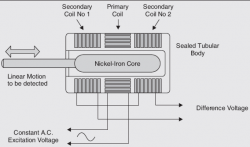# LVDT | Definition, Opration, Application## What is LVDT?

Definition of LVDT Transducer: LVDT stands for “Linear Variable Differential Transducer“. LVDT is a passive differential inductive transducer. This is a variable inductance displacement transducer.

The LVDT is the most common mutual inductance element. This can be considered to be an optimum transducer element for most electro-mechanical measuring systems with regard to resolution, hysteresis, dynamic response, temperature characteristics linearity, and life.

## Construction of LVDT

1. It consists of a primary winding and two identical secondary windings. These windings are axially spaced and bound on a cylindrical coil former.
2. A rod-shaped magnetic core is positioned centrally inside the coil assembly. This rod provides a low reluctance path for the magnetic flux linking the coils (windings).
3. The moving object displacement of which is measured is coupled to this movable rod.
• The two secondary winding are connected in series opposition. Hence the voltages induced into these windings are of opposite polarities.
• The output voltage is given by E0 = E01 – E02
• Where E01 and E02 are the emf induced in two secondary windings.
• The transfer characteristic of LVDT is shown in the figure.
• It is the graph of output voltage against the core position.

## Operation of LVDT

• The primary winding is connected to the ac source.
• Assume that the core is exactly at the center of the coil assembly. Then the flux linked to both the secondary windings will be equal.
• Due to equal flux linkage, the secondary induced voltage is equal, but they have opposite polarities.
• The output voltage of LVDT i.e. E0 is, therefore, zero correspondings to the central portion of the core. This position of the core is called the “null position”.
• Now, if the core is displaced from its null position towards secondary-1, then the flux linked to secondary-2 increases, and flux linked to the secondary-2 to decreases.
• Therefore the induced voltage eo1 is now greater than E02 and the output voltage of LVDT i.e. E0 will be positive.
• Similarly, if the core is displaced downwards i.e. towards the secondary-2 then E02 will be greater than E01 and the output voltage e0 will be negative.
• Thus the magnitude of the output signal is made a very “linearly” with the mechanical displacement. Hence the word linear is used for LVDT.
• The output is obtained “differentially” between the two secondary windings. Hence, the word “differential” is used in LVDT.

1. Linearity: The output voltage of LVDT is almost linear for displacement up to 5mm.
2. Infinite Resolution: The charge in output voltage is continuous, step-less. The effective resolution depends more on the equipment used for the measurement rather than on the LVDT.
3. High Output: LVDT gives reasonably high output and hence requires less amplification afterward.
4. High Sensitivity: LVDT has a high sensitivity of about300 mV/mm: i.e., 1 mm displacement of the core produces an output voltage of 300mV.
5. Ruggedness: This transducer is mechanically rugged and can withstand mechanical shock and vibrations.
6. Less Friction: Since there are no sliding contacts, the friction is very less.
7. Low Hysteresis: It has a low hysteresis; hence its repeatability is extremely good under all conditions.
8. Low power Consumption: Most LVDTs consume less than 1 W of power.
9. This transducer is small, simple, and light in weight. They are stable and easy to align and maintain.

1. LVDT is sensitive to the external magnetic fields. To minimize this effect magnetic shielding is necessary.
2. Complicated circuitry is needed.
3. Due to mass of the core, LVDT is not suitable for dynamic measurement.
4. Large displacements are needed to get appreciable differential output.
5. Temperature affects the transducer.

### Applications

1. It can be used in all applications where displacement ranging from fractions of a few mm to a few cms has to be measured.
2. Acting as a secondary transducer, LVDT can be used as a device to measure force, weight, and pressure, etc. The force or pressure to be measured is first converted into a displacement using primary transducers. Then this displacement is applied to an LVDT, that acts as a secondary transducer, and converts the displacement into a proportional output voltage. In these applications, the high sensitivity of LVDT is a major attraction.

## Recommended Posts

Transducer

Cathode Ray Oscilloscope

## 2 Replies to “LVDT | Definition, Opration, Application”

1.Manual Gudino says:

This web site is known as a stroll-by way of for all the data you needed about this and didn’t know who to ask. Glimpse here, and you’ll undoubtedly discover it.

2. Pingback: Electronics Club